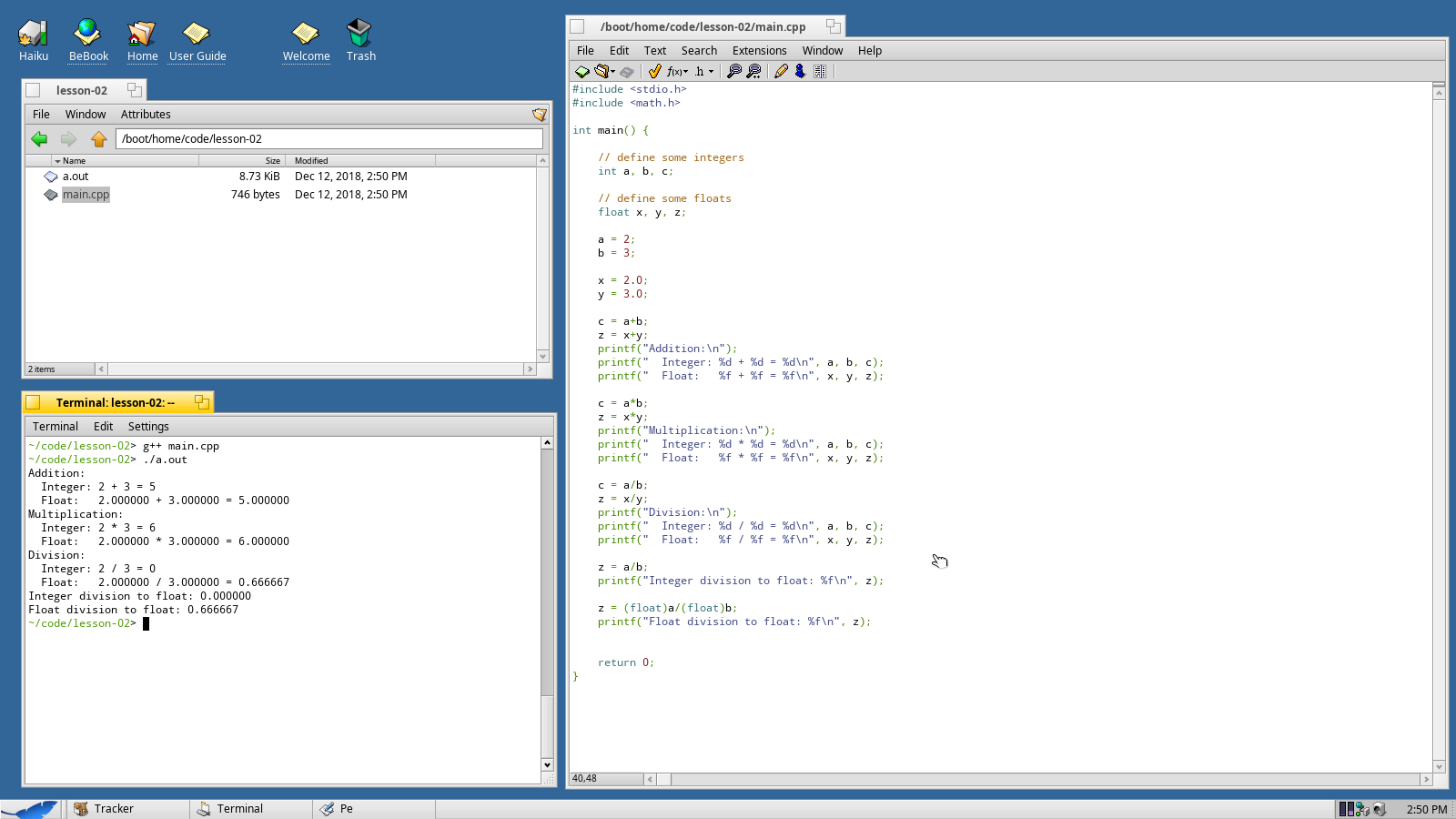Web Developer

# Lesson 2 - Working With Numbers

One of the most basic things you'll do in C/C++ is to store and work with numbers. This lesson will teach you some of the basics for:

• creating a variable
• setting a variable
• doing some math

## Mathematical Concepts

While these tutorials don't assume that you know how to program, it would be helpful if you understood the difference between an integer and a real number.

In elementary school, you may have learned integers as counting numbers and that's somewhat correct - counting numbers are all integers. The only difference is that integers can also be negative.

A real number is an number that can represent a position on a number line - including any position in between any two integers.

## Precision

A lot of real numbers can be represented using a decimal point. ½ can be represented as 0.5, for example. There are, however, some numbers that go on forever (like π) and some numbers that just go on very very far (like 123456789/1000000000, which is the same as 0.123456789).

Although C/C++ can store both integers and real numbers, there are limits to how much space it has to store those numbers.

Precision can vary depending on the computer for which you're compiling. On a PC or Mac an integer can store any number between -2,147,483,648 and 2,147,483,647.

Real numbers can be almost as large as one septillion but they lose accuracy as they get either very large or very small. For numbers that go on a long time (or forever), only the first 23 bits of that number are stored and the rest are just thrown away.

This is like saying that π is about 3.14. We know that it really goes on forever, but most of the time that's close enough for a good estimate.

If you don't remember how to create a project, see Lesson 1. This time, just use a folder called lesson-02.

We're going to start with the following code. Note the new include file `math.h`.

``````#include <stdio.h>
#include <math.h>

int main() {
return 0;
}``````

## Creating Variables

In order to store a number in C/C++ you'll need to create a variable. One important disction for C/C++ is that it's a strongly typed language.

• You must create a varable using a type.
• You cannot change the variable's type later.

Let's create three integer variables - a, b, and c - and three real number variables - x, y, and z. In C/C++ (and most languages) real numbers are referred to as floating-point numbers and from here on out I'll be referring to them as floats.

``````#include <stdio.h>
#include <math.h>

int main() {

// define some integers
int a, b, c;

// define some floats
float x, y, z;

// store some numbers
a = 2;
b = 3;
x = 2.0;
y = 3.0;

return 0;
}``````

Let's practice some basic math now. We'll also learn to output floating point numbers using `printf`.

``````c = a+b;
z = x+y;
printf("  Integer: %d + %d = %d\n", a, b, c);
printf("  Float:   %f + %f = %f\n", x, y, z);``````

You should be able to compile this program and see your first results.Subtraction works exactly the same as addition, so let's skip it for now. Let's move on to multiplication and division instead.

Note that because `x` is a perfectly valid variable name, multiplication uses the asterisk `*` for multiplication.

``````c = a*b;
z = x*y;
printf("Multiplication:\n");
printf("  Integer: %d * %d = %d\n", a, b, c);
printf("  Float:   %f * %f = %f\n", x, y, z);

c = a/b;
z = x/y;
printf("Division:\n");
printf("  Integer: %d / %d = %d\n", a, b, c);
printf("  Float:   %f / %f = %f\n", x, y, z);``````

Compile and have a look at the new results.Multiplication works as you would expect, but division has some odd results. For integer division the result is truncated, meaning that anything after the decimal point is simply thrown away. In this case 0.66666… becomes 0.

Floating point is a little different but because ⅔ goes on forever after the decimal point the floating point number also has to give up after a while. The computer is smart enough however to round up here instead of merely truncating.

## Integer Division and Casting

It isn't totally clear when you look at the code but what's going on behind the scenes is that the computer handles floats and integers in completely different ways. Wholly different parts of the CPU are used to do these operations.

This means that in order to do any math on any number the computer has to have two numbers of the same type. 99% of the time, C/C++ will handle this for you. For the other 1% of the time, you'll need to use casting to change your number's type.

``````z = a/b; // assigning integer division to a float
printf("Integer division to float: %f\n", z);

z = (float)a/(float)b; // casting a and b to floats
printf("Float division to float: %f\n", z);``````## Rounding Numbers

If you try to assign a floating point value to an integer, C/C++ will automatically truncate the number - it'll throw away everything after the decimal point.

There's a function, however, in `math.h` that allows us to round numbers up or down as appropriate. As you've probably guessed, this function is called `round()`.

``````c = x/y;
printf("Truncation on assignment: %d\n", c);
c = round(x/y);
printf("Rounding on assignment: %d\n", c);``````In this case, ⅔ gets truncated to 0, but gets rounded to 1.

## Wrapping Up

In this lesson you learned:

• how to create a variable
• the importance of variable types
• how to do basic math
• how to output floating point numbers
• how to include a new library (math.h) to get access to a function

These are some core principles for working in C/C++ and we'll be building on these concepts going forward.